# Bottomhole Pressure Calculations

Estimating bottomhole pressure from measured wellhead pressure and temperature is an important task in petroleum engineering. Bottomhole pressures are required in most classical well-performance forecasting techniques such as rate-transient analysis (RTA), history matching using reservoir simulation, inflow-performance-relationship (IPR) estimation, and more.

Based on production data measured at the surface (i.e. surface rates, wellhead pressure, and wellhead temperature), and key properties describing the wellbore (i.e. a deviation survey describing the curvilinear shape of the well, wellbore diameter, and pipe roughness), we can estimate the bottomhole pressure by numerical integration of the pressure- and temperature gradient expression.

Multiphase flow in inclined pipe involves calculations of oil, gas, and water which require and accurate fluid description (PVT). We achieve this by leveraging all the relevant capabilities of whitson+ seemlessly to provide accurate and consistent phase behavior in all calculations made on a particular well.

Currently Supported Correlations:

• Hagedorn and Brown (Liquid-Rich Wells)
• Beggs and Brill (Liquid-Rich Wells)
• Gray (Low-LGR Gas Wells)
• Woldesemayat and Ghajar (Liquid-Rich Wells)

## 1. Input

### 1.1 Production data

Daily production rates can be provided at stock-tank conditions or at separator conditions. If separator rates are provided, then the Common Process Conversion module can be used to generate stock-tank rates for the BHP calculations. In addition to production rates, one must also provide casinghead pressure and tubinghead pressure if a tubing is installed. If the well is subjected to either gas-lift or rod-pump assisted lift, lift-gas rates or liquid level in the annulus must be provided, respectively.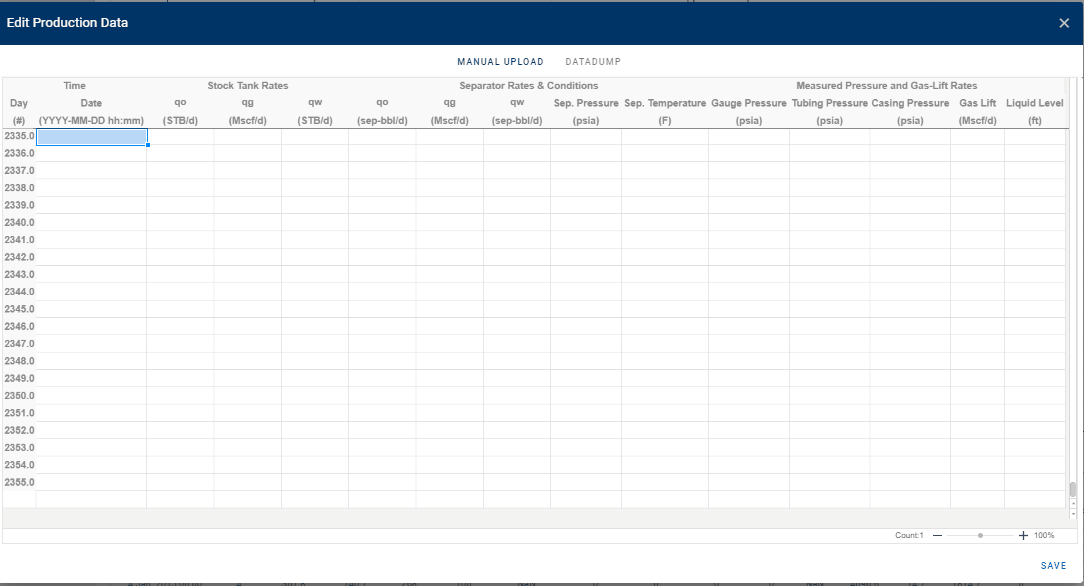### 1.2 Well deviation survey

The well deviation survey describes the trajectory of the wellbore, and consists of a measured depth (MD) and calculated true vertical depth (TVD). The user can provide the full deviation survey of the well as the bhp module will sample the deviation survey backend to create the wellbore grid for BHP calculations. The perforated interval must be specified by providing the MD of the top- and bottom perforations. The top perforation is also the reference depth at which the BHP is calculated.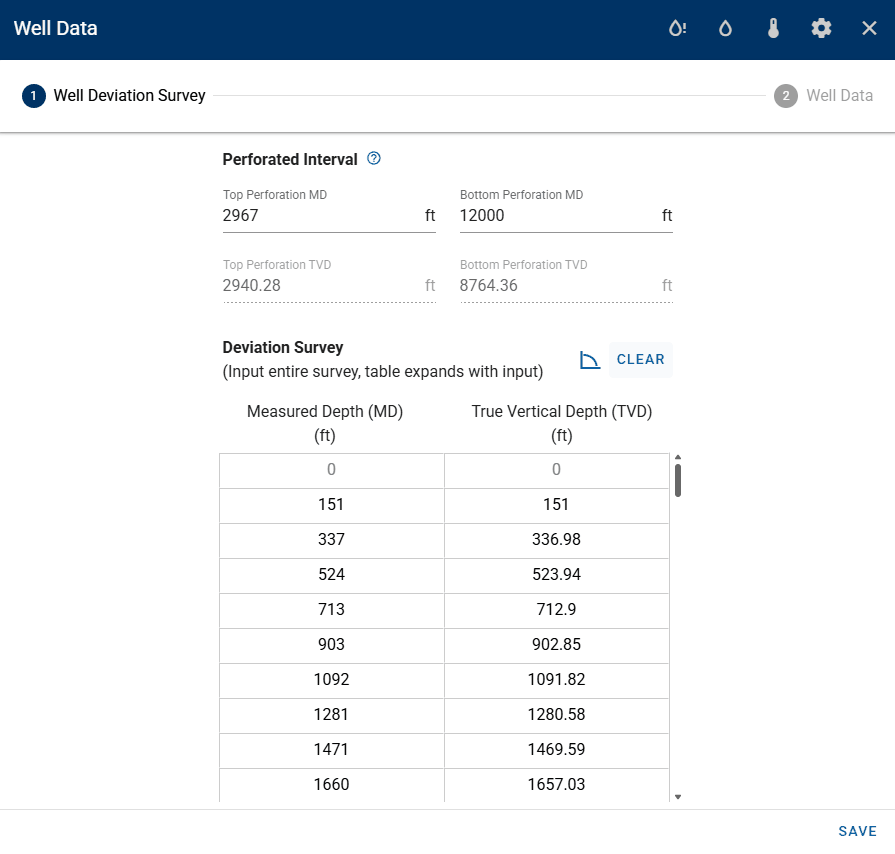### 1.3 Well data

#### 1.3.1 Casing- and Tubing Data

The well data consist of properties for the tubing- and casing string(s) relevant for pipe flow calculations, (i.e. the depths of the different pipes, their diameters, and roughnesses). Multiple tubing and casing strings can be specified, where an API tubing- and casing table is available to simplify the input of the pipe data.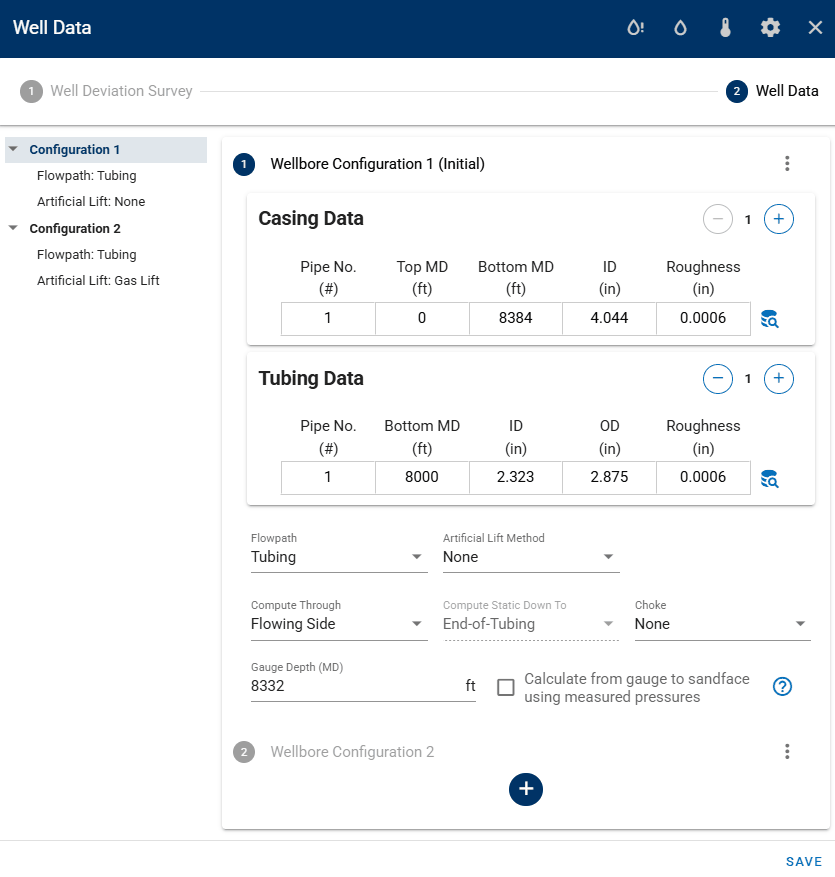Multiple completion data sets can be specified, where a date must be set to tell the bhp module from which date in the production time series each completion is used.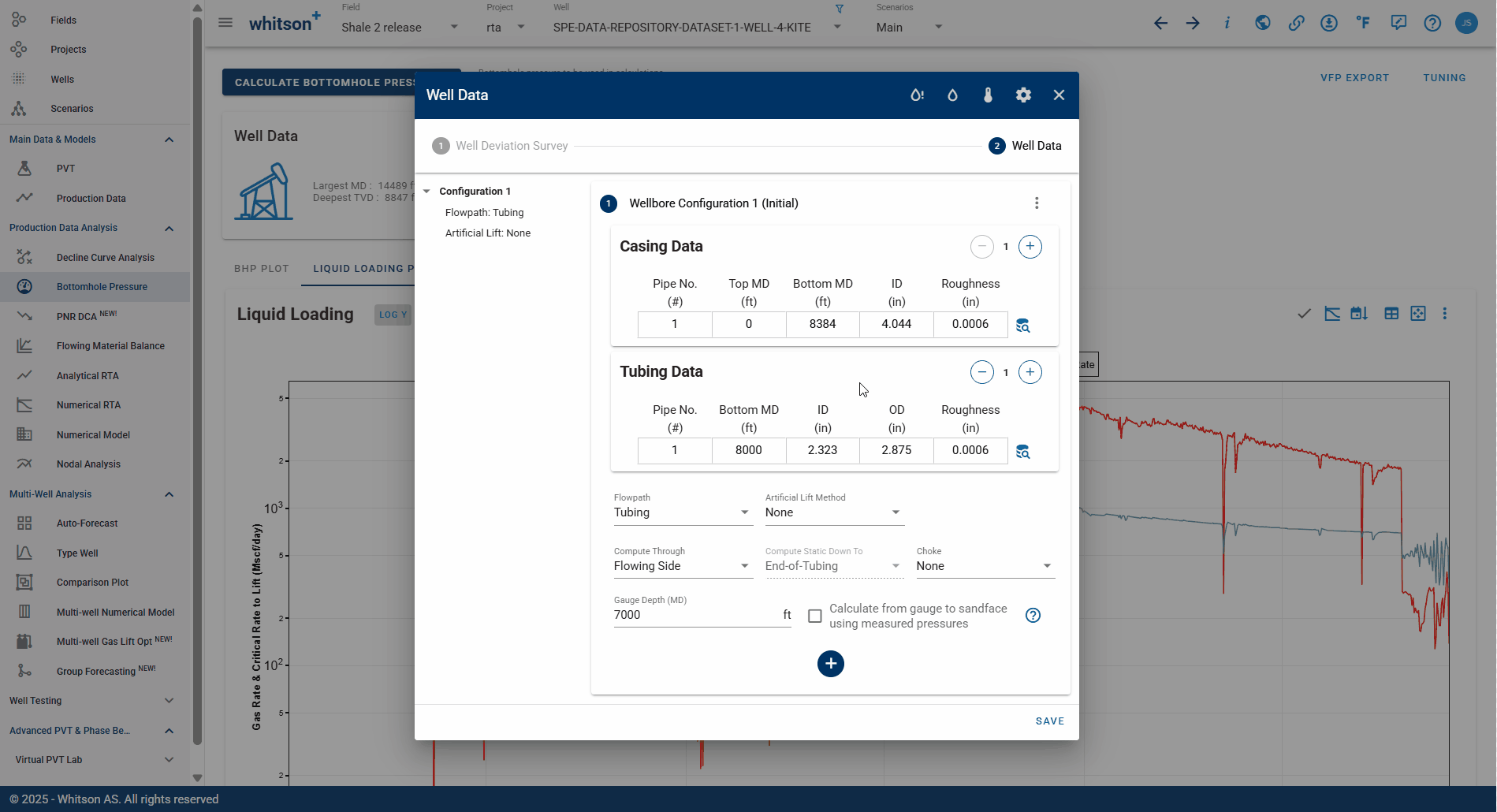#### 1.3.2 Flow Paths

The user must also specifiy the flow path of the wellstream for each wellbore configuration. The flow path can be:

• Tubing—Wellstream flows through a tubing string
• Casing—Wellstream flows through the production casing
• Annulus—Wellstream flows in the annulur space between the tubing and production casing
• Tubing & Annulus—Wellstream flows both through the tubing string and the annulur space between the tubing and production casing. See the section on dual conduit flow for further details.
• Measured BHP—If pressures from a downhole gauge have been provided under the Gauge Pressure column in production data, one can instruct the BHP model to echo the measured BHP (applies to wells for which an accurate estimate of the BHP is available, and where the user wants to include that pressure in the resulting BHP profile for completeness)
• Unknown—Automatic selection of flow path depending on the casinghead pressure, tubinghead pressure, and associated well configuration. The following table summarizes the logic applied for the unknown flow path.

Condition Selected Flow Path
$$p_{casing}$$ $$>$$ $$p_{tubing}$$ and Tubing is Installed Tubing
$$p_{casing}$$ $$\leq$$ $$p_{tubing}$$ and Tubing is Installed Annulus
Tubing is not Installed Casing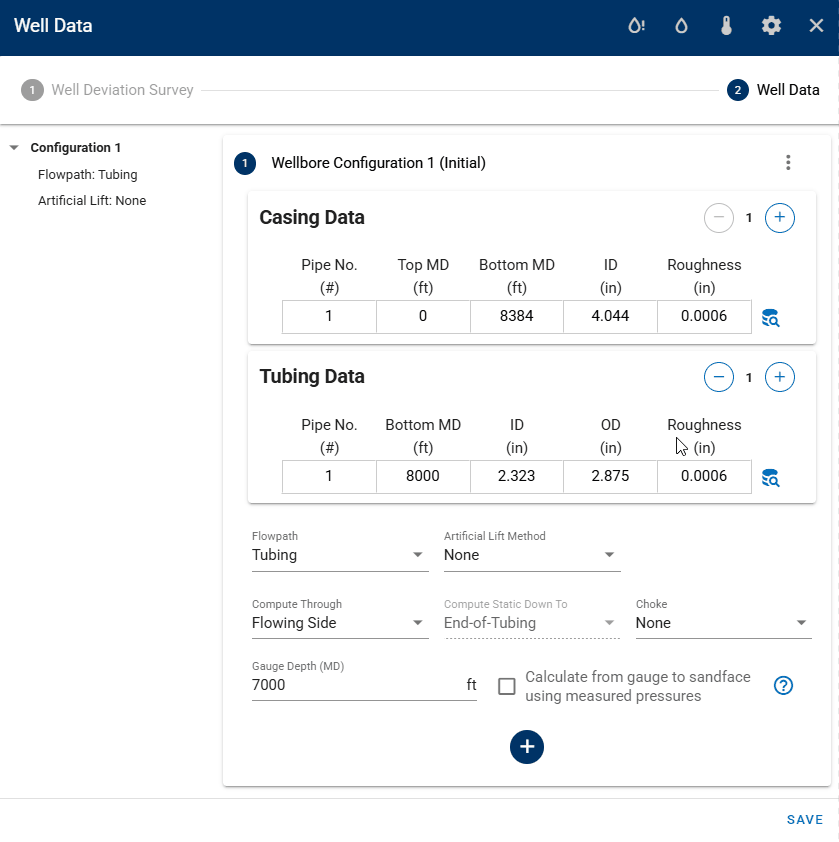#### 1.3.3 Static (Quiet) Side

The user may select which side of the tubing the bottomhole-pressure calculation should follow. Using the static (quiet) side of the tubing assumes that there is a single-phase gas column with communication to the flowing side at the EOT (i.e., no isolating packer).

The static-side calculation is well suited for gas-lift assisted wells where it is certain that the static side is filled with gas at least down to the gas-lift valve. The figures below explain how the static-side calculation works for a naturally flowing well, and a gas-lift assisted well. The flow path in this case is set to "tubing", but the static-side calculation also works when the flow path is set to "annulus", with everything being reversed compared to the figures below.

For gas-lift assisted wells where the gas-lift configuration is set to "valves" or "automatic", it is also possible to select whether to do the static-side calculation down to the gas-lift valve or to the end of the tubing.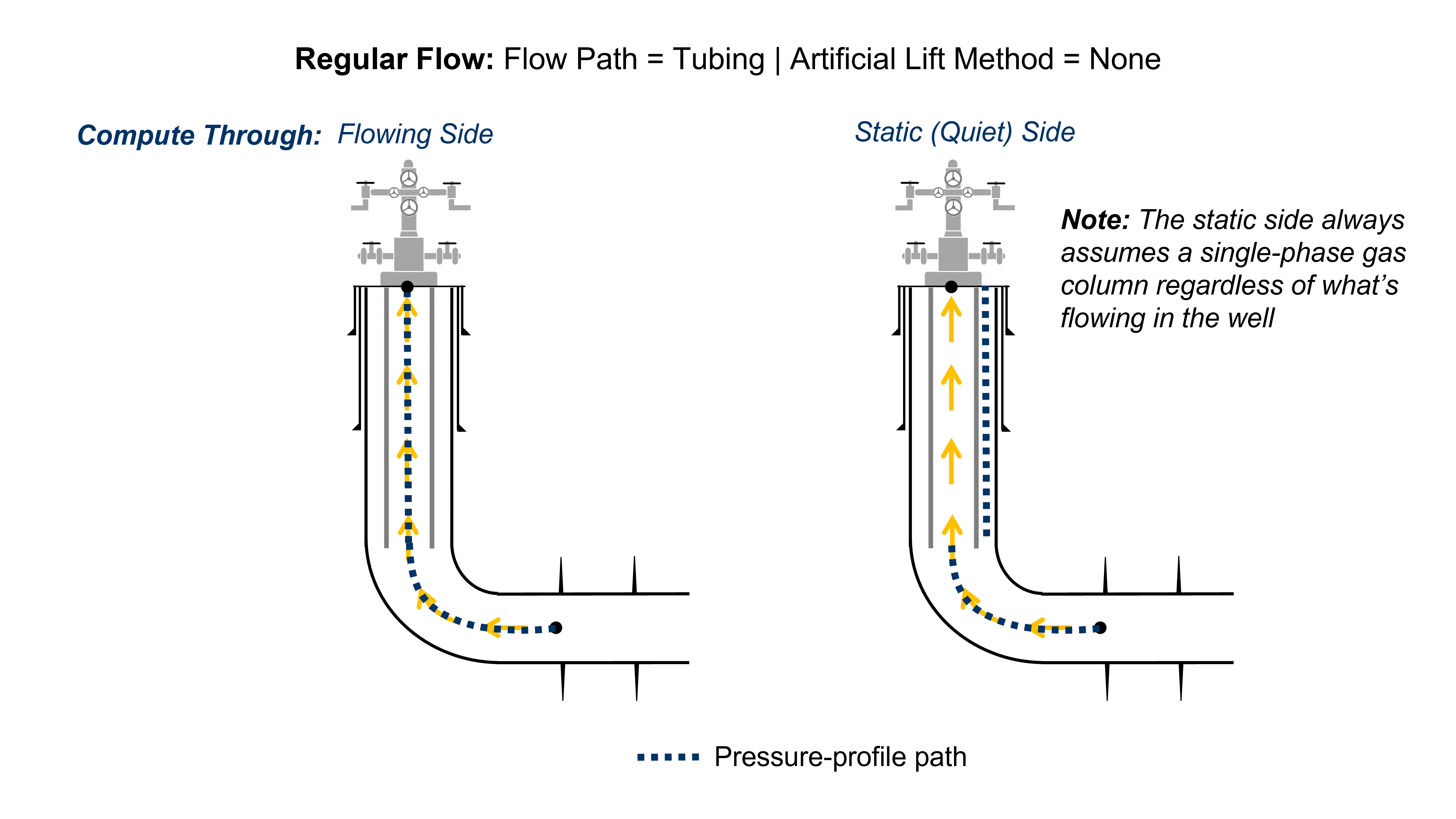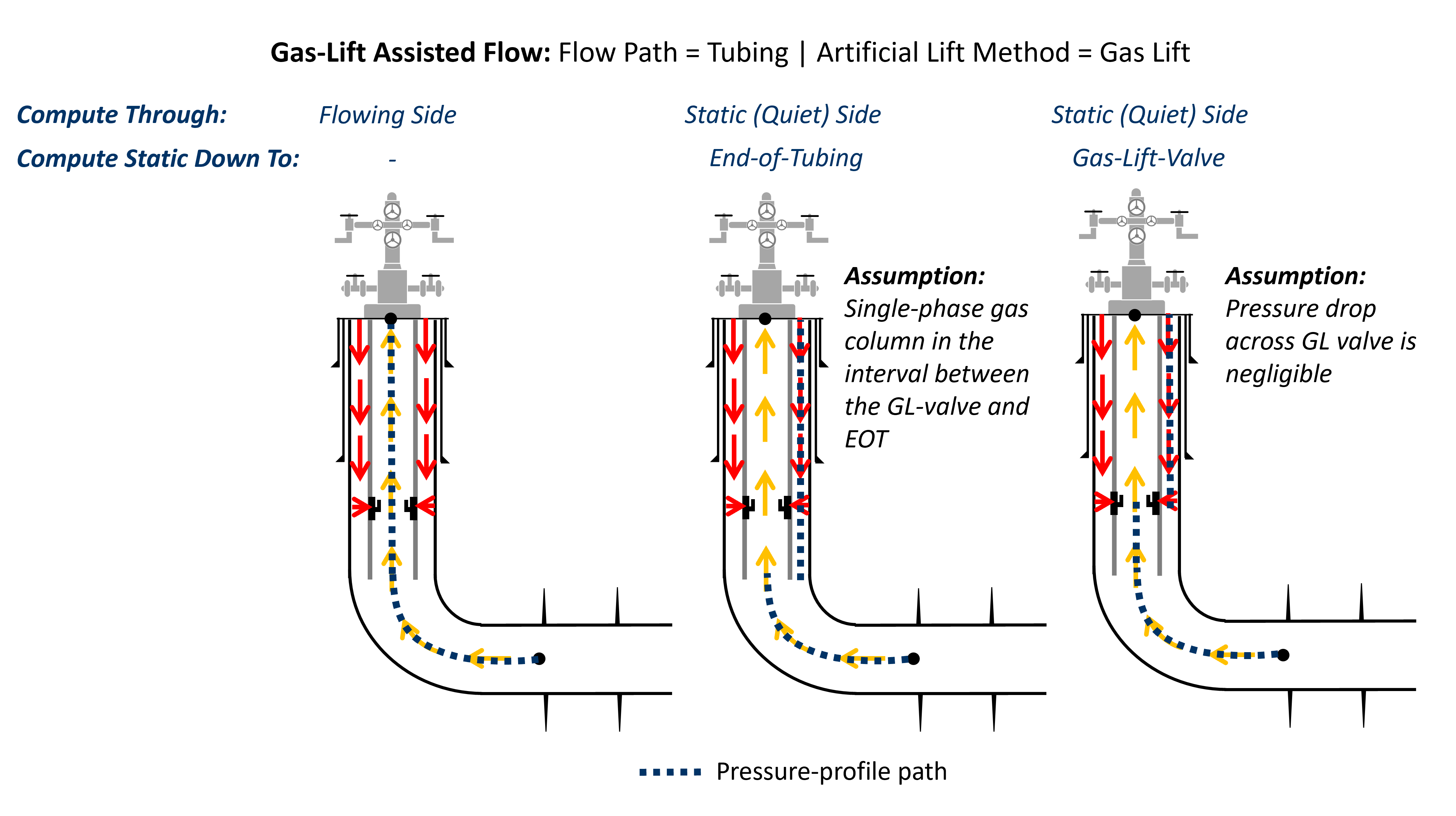#### 1.3.4 Convert from Gauge Pressure to Sandface

If the user has entered pressures for one or several rows in the Gauge Pressure column in production data, one can convert from this pressure and down to sandface. To do this, toggle on "Calculate from gauge to sandface using measured pressures".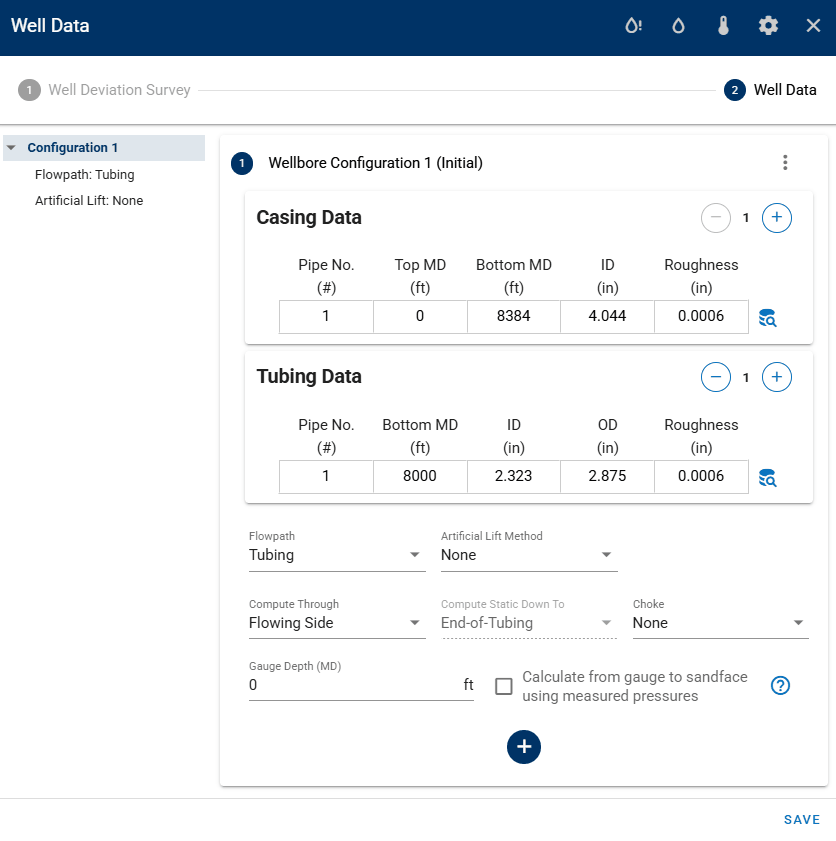### 1.4 Temperature Data

The temperature data require two inputs, the rock temperature at the surface (to create the linear temperature profile in the rock), and the average wellhead temperature for the production time series.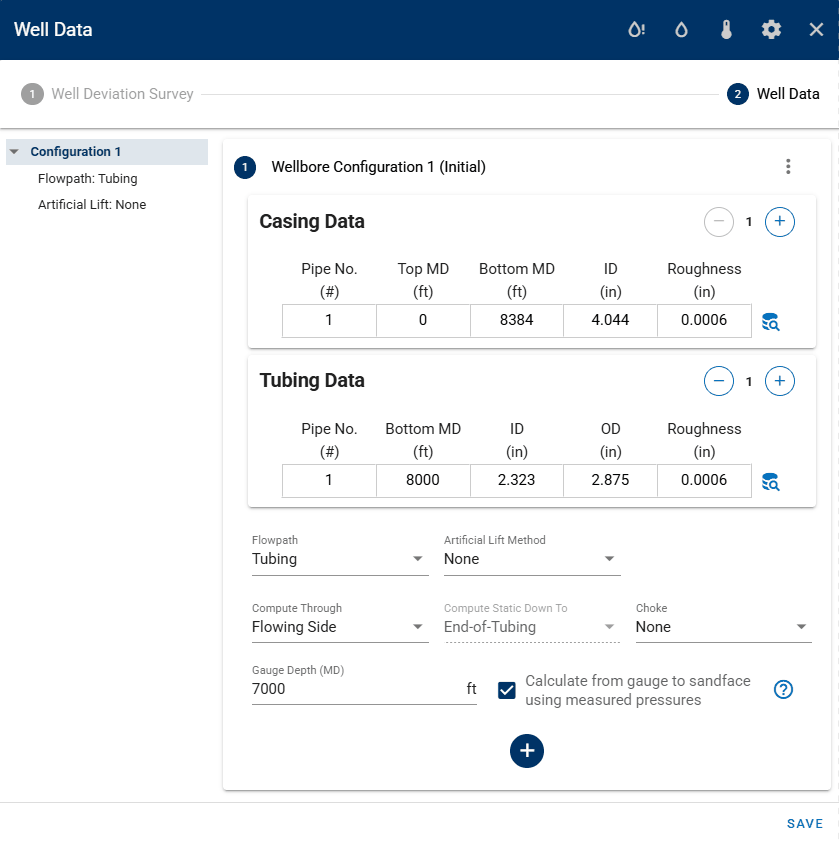### 1.5 Artificial-Lift Data

The BHP can be calculated while the well is on gas-lift, rod pump or plunger lift.

Gas lift is active for a given day if the active wellbore configuration for that day has gas lift as the artificial lift method, and $$q_{g,gas lift}$$ in the production data is greater than zero.

Rod pump is active for a given day if the active wellbore configuration for that day has rod pump as the artificial lift method, and the "Liquid Level" in the production data is greater than zero.

Plunger lift is active for a given day if the active wellbore configuration for that day has plunger lift as the artificial lift method, and the "Liquid Level" in the production data is greater than zero.

ESP (Electric Submersible Pump) can only be activated if downhole pressures are measured at the inlet of the ESP with a pressure gauge. If this option is selected, the user can provide the depth of the pressure gauge, and correct the pressures from measurement point down to sandface.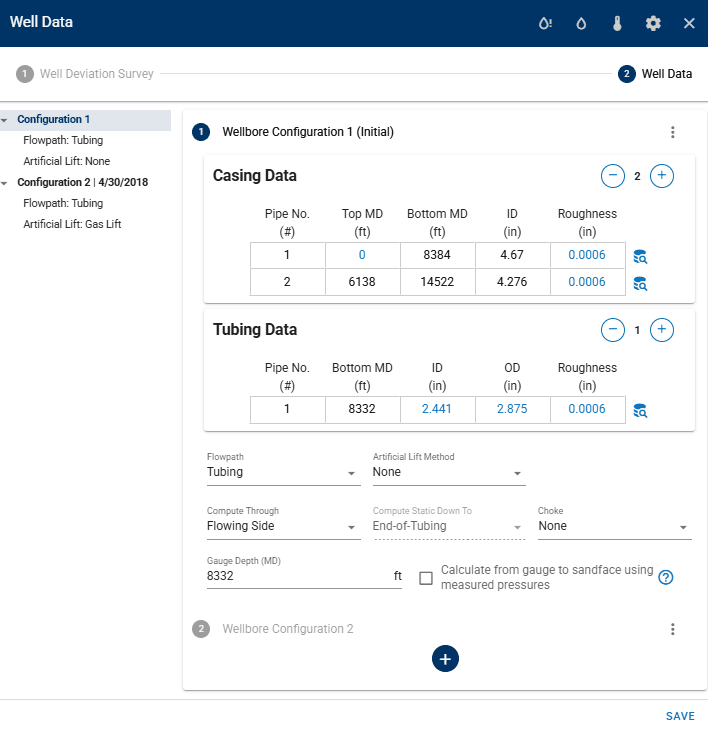## 2. Output

### 2.1 Output Curves

The output data for the BHP module is the calculated bottomhole pressure, at the top perforation depth, for every entry in the production time series. The user can choose whether to use the noicy data resulting from noicy production data, or to apply a smoothening of the data. Furthermore, the user can select which BHP data set to use in other features of whitson+ that rely on bottomhole pressures. This ensures consistency in all calculations across the platform.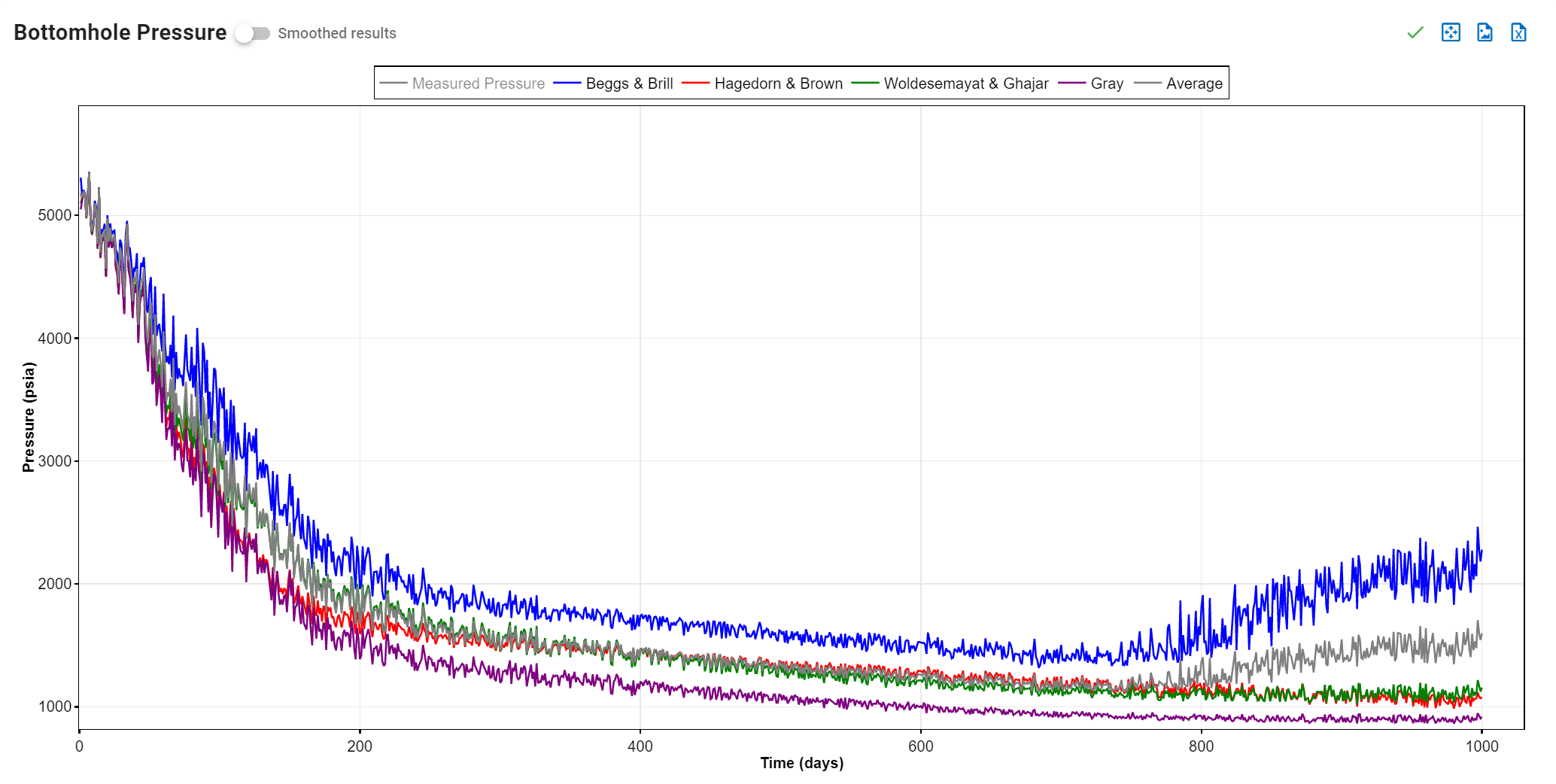### 2.2 Custom BHP

Calculated bottomhole pressures are generally noisy due to oscillations in surface rates and pressures. This noise in the bottomhole pressures can have a large impact on the analysis being performed, specially RTA / numerical modeling when the well is controlled on bottomhole pressures. With the custom BHP feature it is possible to piecewise-linearize and smooth the calculated bottomohole pressures. The user can select the sampling frequency and also manually add or remove points from the chart to create the shape / curve that is desired.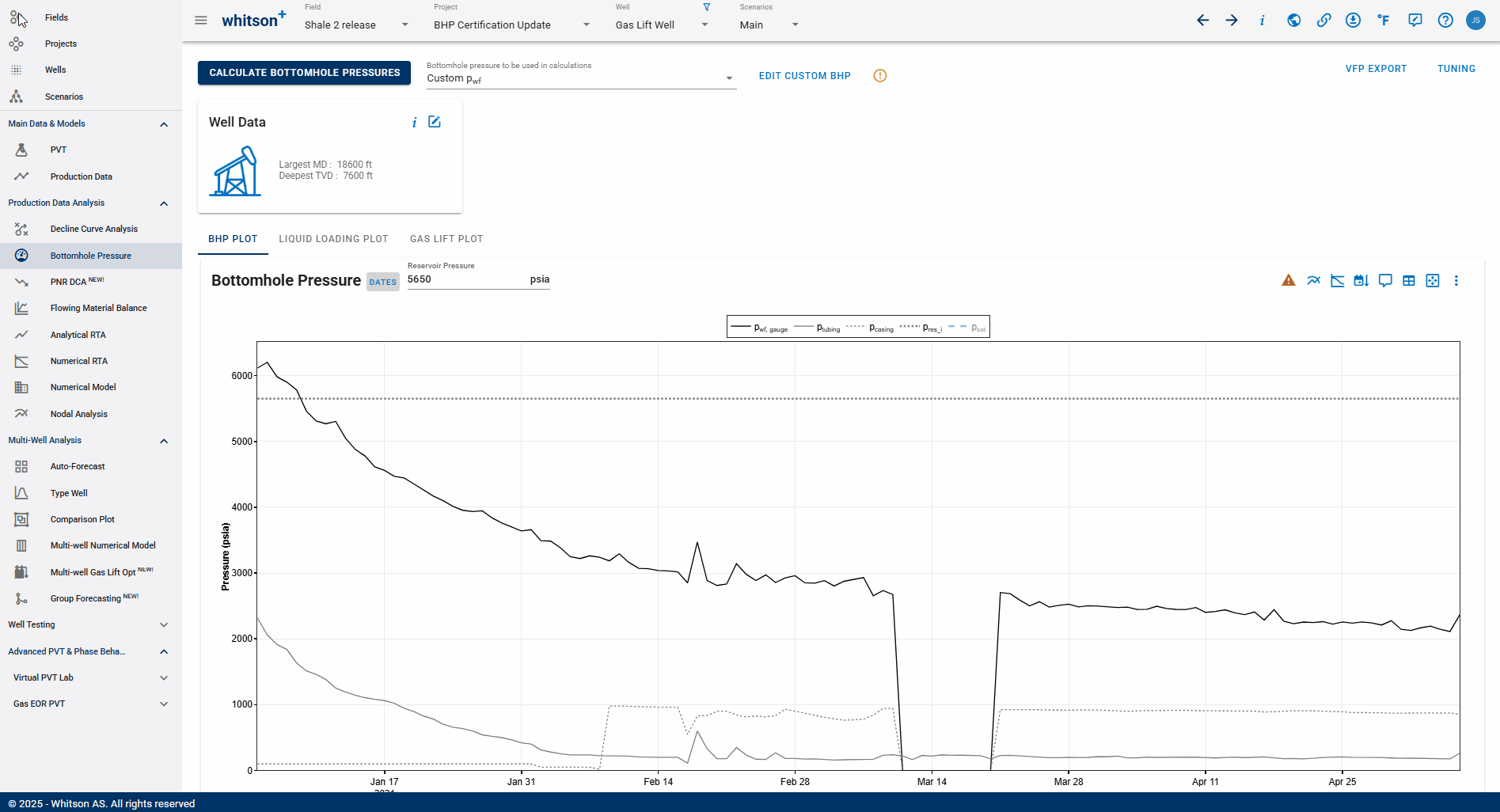### 2.3 BHP Calculation Stuck?

When the computation takes longer than expected, the user can try to force re-running the calculation as follows:

1. Open Well Data card
2. Click Calculation Options
3. Click refresh icon to Force Run BHP Calculation
4. All steps are shown in the .gif below.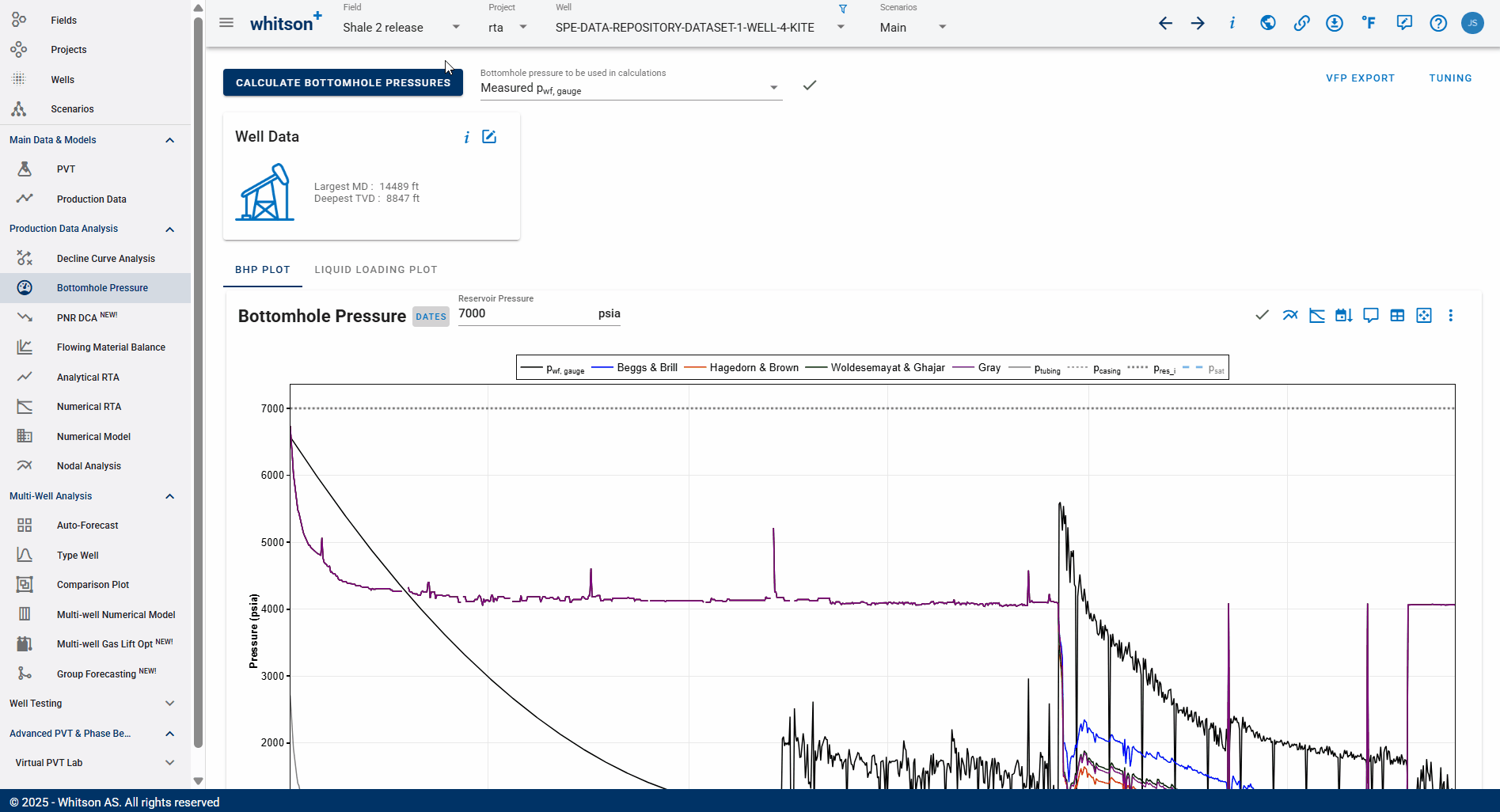## 3. Technical Features

### 3.1 Governing Equations

The main equations used for multiphase flow in inclined pipe are provided here. Our wiki contains a detailed review of the relevant multiphase-flow theory and the commonly used correlations for pipe flow.

### 3.2 Artifical-Lift Methods

#### 3.2.1 Gas Lift

Gas lift is included as a step change in mass flow at the injection valve. We assume the lift gas to completely mix with the flowing mixture, resulting in a step change in the gas rate, and consequently the producing GOR.

When the well is on gas lift, are gas rates netted or gross (including gas lift rates)?

The inputted gas rates in the production data editor are assumed to be netted out

The reason we do this is because the gas rate is used everywhere in the system, including numerical model, flowing material balance and RTA; and there the gas lift is not part of the calculation (i.e. not part of the reservoir flow).

In the BHP calculations, to get the total gas that is flowing inside the wellbore, we just do the calculation in the background as follows:

Three options exist for the gas-lift configuration.

Poorboy: The term "poorboy" refers to the situation when injection occurs at the EOT.

Valves: A single valve at a specified depth, or a set of valves at different depths along the tubing, can be provided. If a full set of valves is provided, the casinghead pressure is used to decide which valve the lift-gas enters the tubing through. We assume all of the lift gas to enter the tubing through the first open valve it encounters, even though this rate might exceed the maximum rate predicted by the Thornhill-Craver equation. Note: The gas lift valves are ordered by descending measured depth with valve number. The deepest valve, is valve number 1, the second deepest is valve number 2, and so on. If "Last Valve Always Open" is ticked, then PSC and PSO for valve 1 (if specified) will be disregarded by the calculator.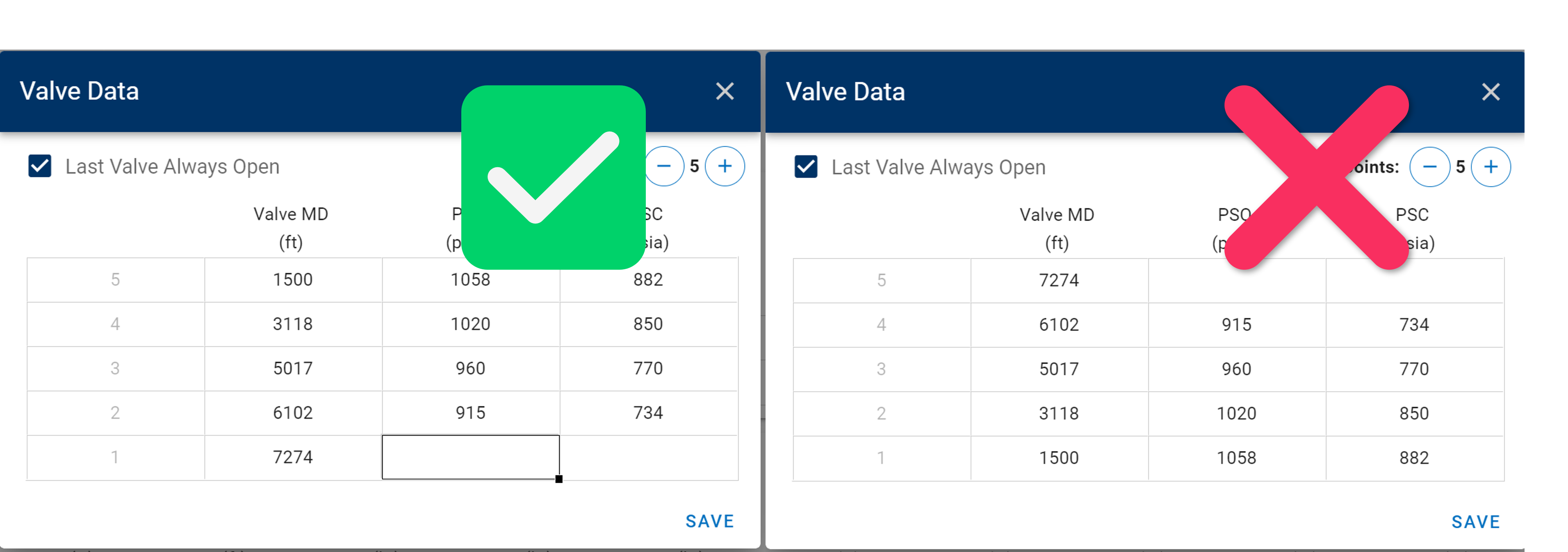Automatic: If the lift-gas injection depth is unknown, then the BHP calculator can estimate the depth by computing the pressure profiles in the tubing and annulus and finding the depth at which they are equal. If this depth is computed deeper than the EOT depth, then EOT depth is used (poorboy).

If the flow path for a given day is set to "Annulus", a reversed gas-lift configuration is applied where the point of injection is set to be at the deepest valve if there is only a single valve depth specified or "Last Valve Always Open" is ticked for a set of valves. If not, the lift-gas is injected at the EOT.

Which gas lift configuration to choose for a given well?

Is there a packer in the well? If so, it is likely that the gas lift configuration uses valves, meaning that the injected gas enters through valves in the tubing. In this case the depth of the valve(s) that allow gas to flow from annulus to tubing (or viceversa) is a required input.

If packers are in the hole, but the valve depth is unknown, then picking the “Automatic” gas lift configuration is the best choice, then the BHP calculator can estimate the depth by computing the pressure profiles in the tubing and annulus and finding the depth at which they are equal (that would be the injection depth). One important consideration is that using "Automatic" gas lift configuration in this case might lead to the injection happening at end of tubing (EOT), which would not be consistent with having a packer in the whole, but this will only occur if the depth is computed to be deeper than EOT based on the input data.

If there is no packer installed in the hole, there is communication between annulus and tubing through the end-of-tubing (EOT) and therefore the appropriate gas lift configuration is “Poor-boy”.

#### 3.2.2 Rod Pump

BHP calculations with rod pump applied is achieved by dividing the well into three parts:

1. Wellhead to Liquid Level—Gas flowing in the annulus

2. Liquid Level to Inlet of Pump (EOT)—Static fluid column in the annulus

3. Inlet of Pump (EOT) to Top Perforation—Full wellstream flowing in production casing

We assume the fluid split at the inlet of the pump to be such that all surface gas goes through the annulus, and all liquid goes through the pump and up the tubing. This is an approximation of the true split, and thus the estimated BHP may be somewhat larger than the true solution (less gas goes through the annulus in real life than what is modeled).

Most of the pressure drop for rod pump occurs in the second part, (i.e., the static column of fluid) as this is most-often along the vertical part of the well where the gravity pressure drop dominates. This fluid column will in most cases be liquid-like where the liquid is stagnant and the produced gas is percolating/rising through the liquid as bubbles. A new model to honor this liquid-like fluid column has been introduced to the rod-pump calculations, as the original model was overly sensitive to gas rate and consequently predicted too low pressure drop in the fluid column.

The figure below show four flow regimes/modes for rod pump.

• The flow regimes to the left represents the situation when the gas is not able to carry the liquid with it, causing a well-defined liquid level in the annulus. This model has been implemented, with a critical liquid hold-up set to 0.7.

• The flow regimes on the right represents the situation when the gas is able to carry liquid with it. This is not implemented yet.

Unifying the left and right flow modes requires (1) a method of estimating the critical liquid hold-up (i.e., the transition between left and right models), and (2) a method of estimating the fraction of the total produced liquids that goes up the annulus. This is under development.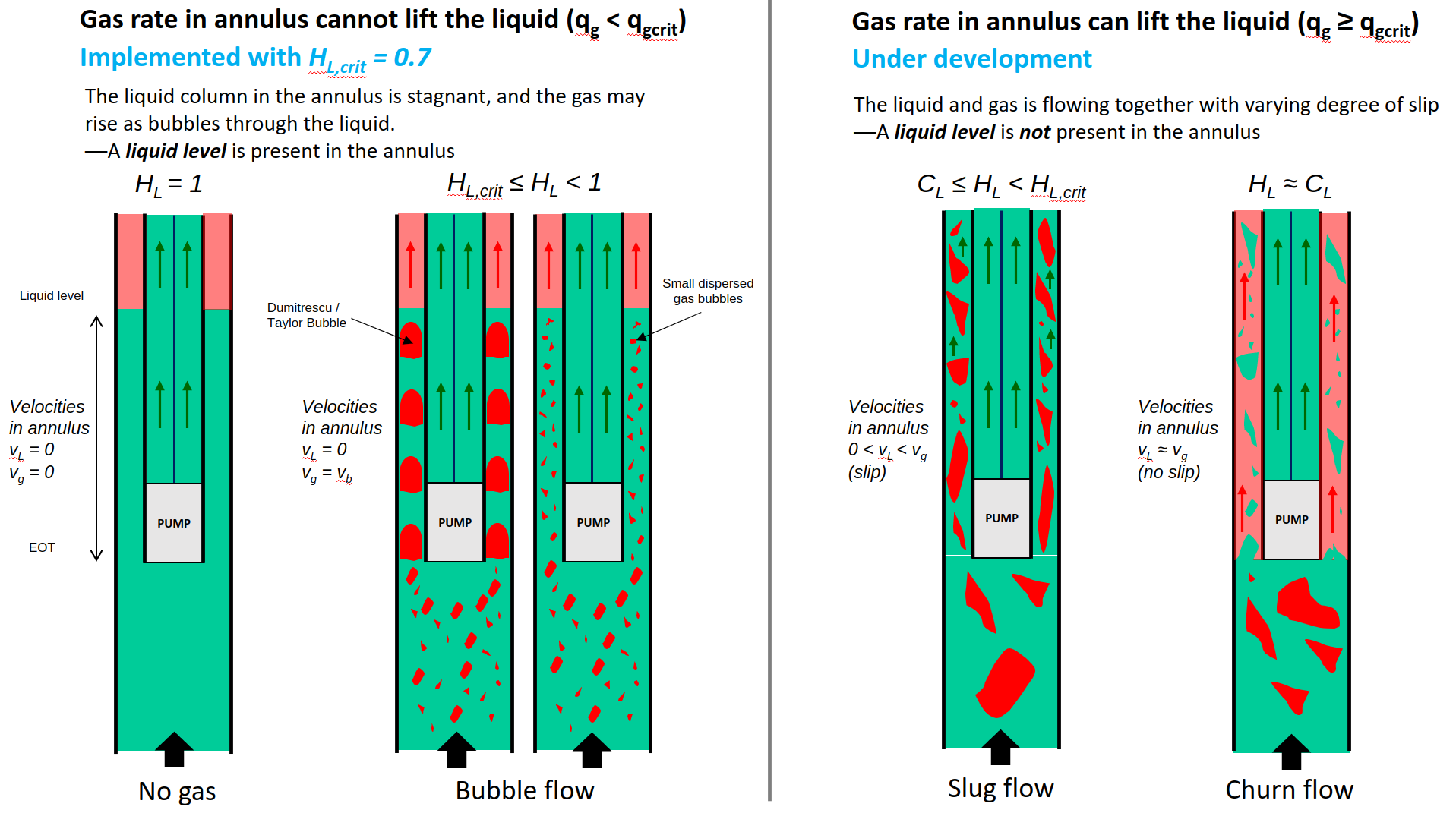#### 3.2.3 Plunger Lift

Plunger lift is a method of artificial lift that uses a free piston traveling up and down inside the tubing in a cyclic manner. When traveling up, the piston removes accumulated liquids from the wellbore which reduces flowing bottom-hole pressure and increases flow rates for a period. When there is liquid accumulation again, the well is shut-in, the piston travels down and the process is repeated.

When a well is operating on plunger lift, the wellbore flow is constantly going through short flow transients due to the cyclical nature of plunger lift operation. To calculate bottomhole pressure in these cases would require a small time scale simulation of the transient process and that would be outside the scope of what we normally want to do for practical petroleum engineering applications. However, it is still possible to calculate an average steady-state bottomhole pressure for plunger lifted wells as follows:

1. If liquid level is measured in the annulus, use the Rod Pump method and provide a liquid level together with the production data. Make sure the liquid levels input correspond to times when the well is producing.

2. Calculate the bottomhole pressures as if plunger was not installed by using the average rates of a producing time period. To capture the effect of less liquid in the tubing during the production period, it is also possible to reduce the OGR of the well.

#### 3.2.4 Jet Pump

A jet pump consists of four static parts: nozzle, throat inlet, throat and diffuser. Highly pressurized power fluid is converted to kinetic energy through the nozzle. Reservoir fluid, with a much lower pressure, is accelerated into the throat. The fluids are mixed in the throat and a homogeneous mixture is developed at the diffuser inlet. The kinetic energy of the mixed fluids is converted to pressure energy in the diffuser. This pressure increase across the pump is what lifts the mixture to the surface.

It is not possible to calculate bottomhole pressures in whitson+ when the wellbore has a jet pump installed. Jet pumps are one of the most difficult pumps to model for hydraulic calculations, and a lot of the information needed to make the calculation is proprietary of the owner of the pump. That is why most pump suppliers have their own software for modeling hydraulics with jet pumps.

In addition to the geometrical configuration and size of the different jet pump components, the most important jet pump parameters that are generally held confidentially by the jet pump supplier are the loss coefficients:

• Nozzle loss coefficient
• Suction loss coefficient
• Throat loss coefficient
• Diffuser loss coefficient

Recommendation for Well with Jet Pump. It is possible to have a bottomhole pressure gauge installed while operating with jet pump, and that is the best way to estimate the flowing bottomhole pressure in such cases. An alternative to finding the correct bottomhole pressures for a period with jetpump is to use the custom pwf feature in whitson+, and “draw” a pressure profile in the jet pump period that is more aligned with an expected pwf decline for the given well. This exercise is even better if you have pwf before and after the jet pump was installed, so we are confident in the limits of the interval (and the drawing is not just a shot in the dark).

### 3.3 Dual-Conduit Flow

If the flow path is set to "Tubing and Annulus", the BHP is calculated by allowing the wellstream to flow in through both annulus and tubing. The split of flow must be solved for by requiring that the pressure at the end of tubing is the same when calculating the pressure drop through both conduits, starting with casinghead pressure for the annulur flow, and tubinghead pressure for the tubing flow.

The Turner or Coleman rates can be used to estimate if the well is potentially liquid loading at any point in time during the production history. A warning will be displayed to the user if the well is potentially loading.

A typical signature of a well experiencing liquid loading is when the gas rate drops without a change in the surface recorded pressures.

### 3.5 Correlation Tuning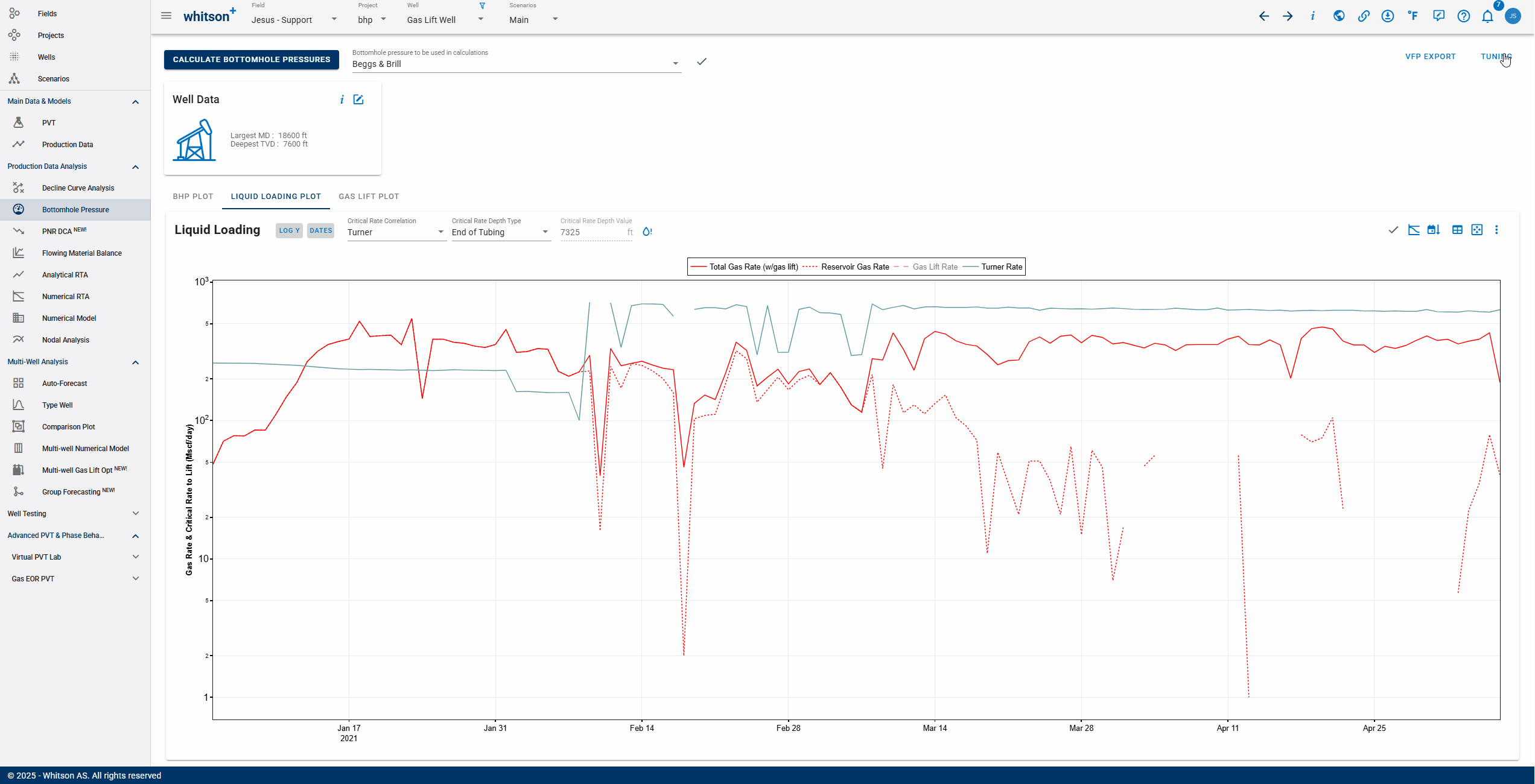The BHP tuning functionality can be accessed by clicking Tuning to the upper right of the BHP feature as seen above.

#### 3.5.1 Correcting the Properties

The multiphase-flow correlations used to compute the bottomhole pressure can be tuned in whitson+. This is achieved by correcting the liquid hold-up by a parameter $$\alpha$$, and the friction factor by a parameter $$\beta$$. Correcting the liquid hold-up affects mainly the gravity pressure drop, while correcting the friction factor affects the friction pressure drop. The corrections are made as follows:

Liquid Hold-Up Correction:

The liquid hold-up, $$H_L$$, is always lower bounded by the liquid flux fraction, $$C_L$$, and upper bounded by 1. To honor this bracketing in a tuning process, it is easier to convert the liquid hold-up into an equivalent slip velocity that is only lower bounded by 0, and then apply the $$\alpha$$ parameter as a correction multiplier. The slip velocity is defined as

where $$v_g$$ and $$v_L$$ are the gas and liquid velocities, and $$v_{sg}$$ and $$v_{sL}$$ are the superficial gas and liquid velocities. Solving (\ref{eq:slipVelocity}) for $$H_L$$, we get:

The liquid hold-up correction is made by

1. Compute the slip velocity $$v_s$$ from $$H_L$$ predicted by the default/untuned correlation using Eq. (\ref{eq:slipVelocity})
2. Multiply the slip velocity by $$\alpha$$ to get $$v_{s}^{corr}=v_s\alpha$$
3. Calculate $$H_L^{corr}$$ with the corrected slip velocity $$v_s^{corr}$$ using Eq. (\ref{eq:liquidHoldUp})

Friction-Factor Correction:

The friction factor is corrected by multiplying the calculated friction factor from the default/untuned correlation by $$\beta$$.

#### 3.5.2 Selecting the Measured Pressure Points to Tune

The tuning procedure includes a routine that selects a subset of the measured gauge data over time. This is done for two reasons, (1) to speed up the tuning process by computing less pressures points, and (2) to minimize the effect of outliers. The sampling procedure involves three steps:

1. Remove outliers that has more than a 20% relative difference from its neighbors.
2. Compute a moving average of the filtered data
3. Sample the moving average at a constant spacing of 20 days.

#### 3.5.3 Correlation Tuning Using Measured Pressures from an ESP

Measured pressures from an electric submersible pump (ESP) should not be used to tune the bottomhole-pressure correlations. The reason for this is that the computed pressures from wellhead to gauge (situated at the ESP inlet) does not account for the delta-pressure caused by the ESP.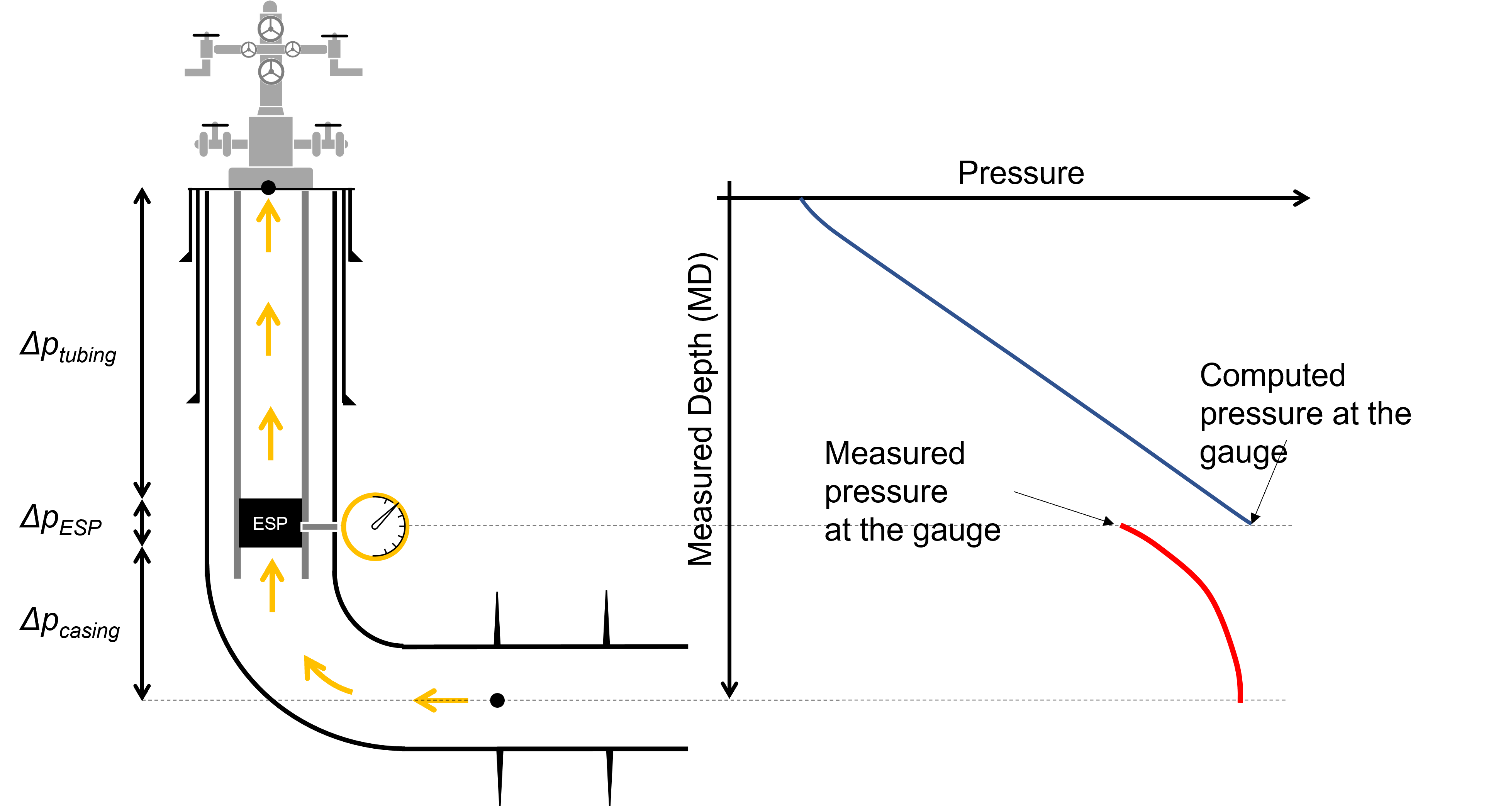### 3.6 Activate BHP Injection Mode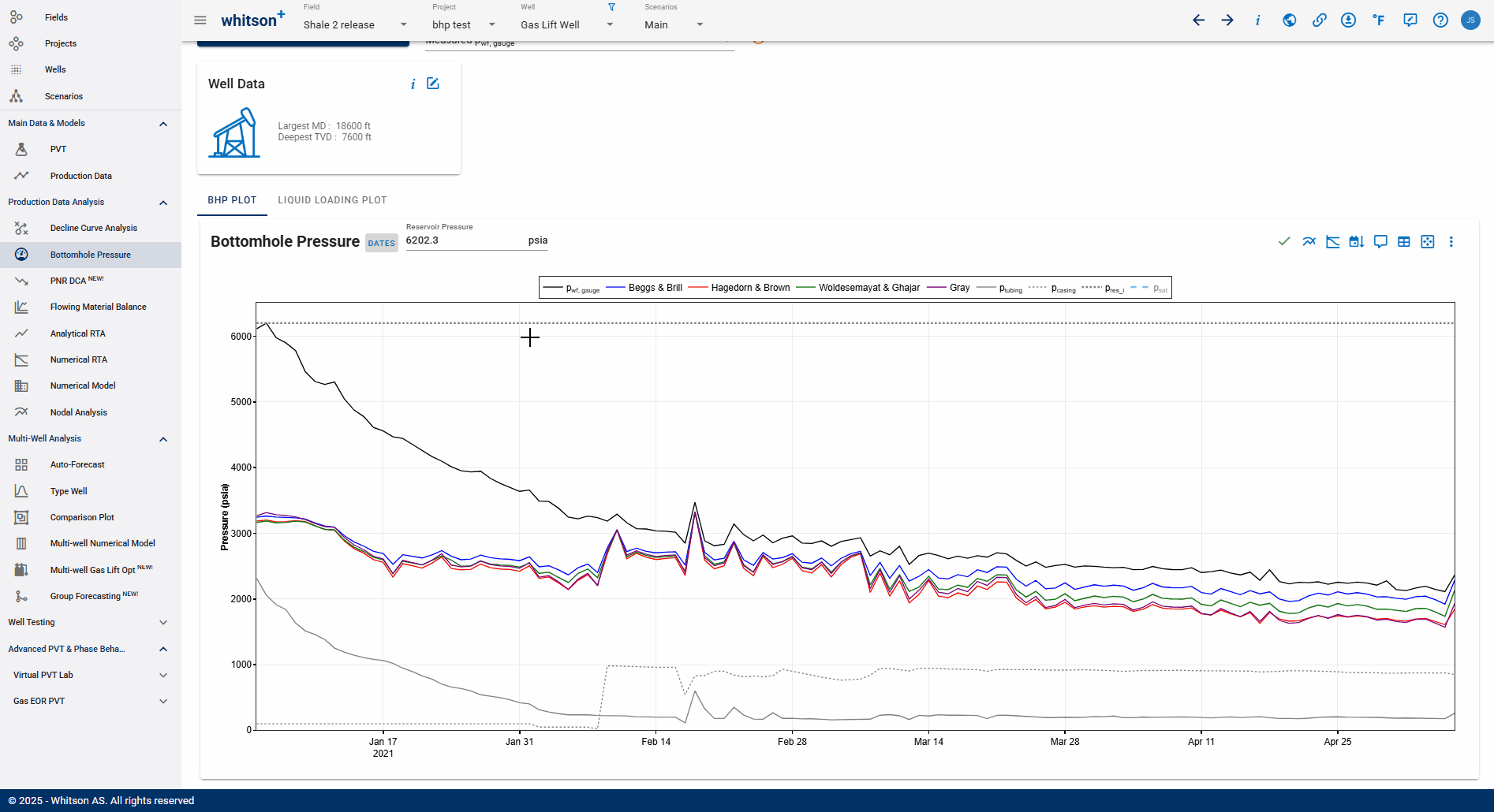If you want to model the bottomhole pressure during an injection process, that can be done by activating the BHP injection mode. This is done in the Calculation Settings in the Well Data card as seen above. When enabled, this switch allows to simulate the pressure at the bottom of the wellbore during injection. All uploaded rates (e.g. oil, water or gas) will be assumed to be injected rates.